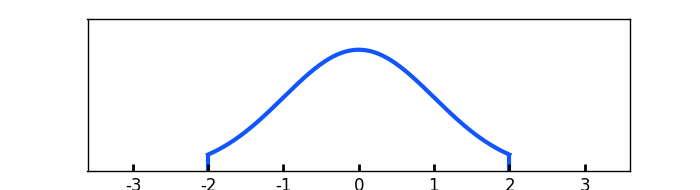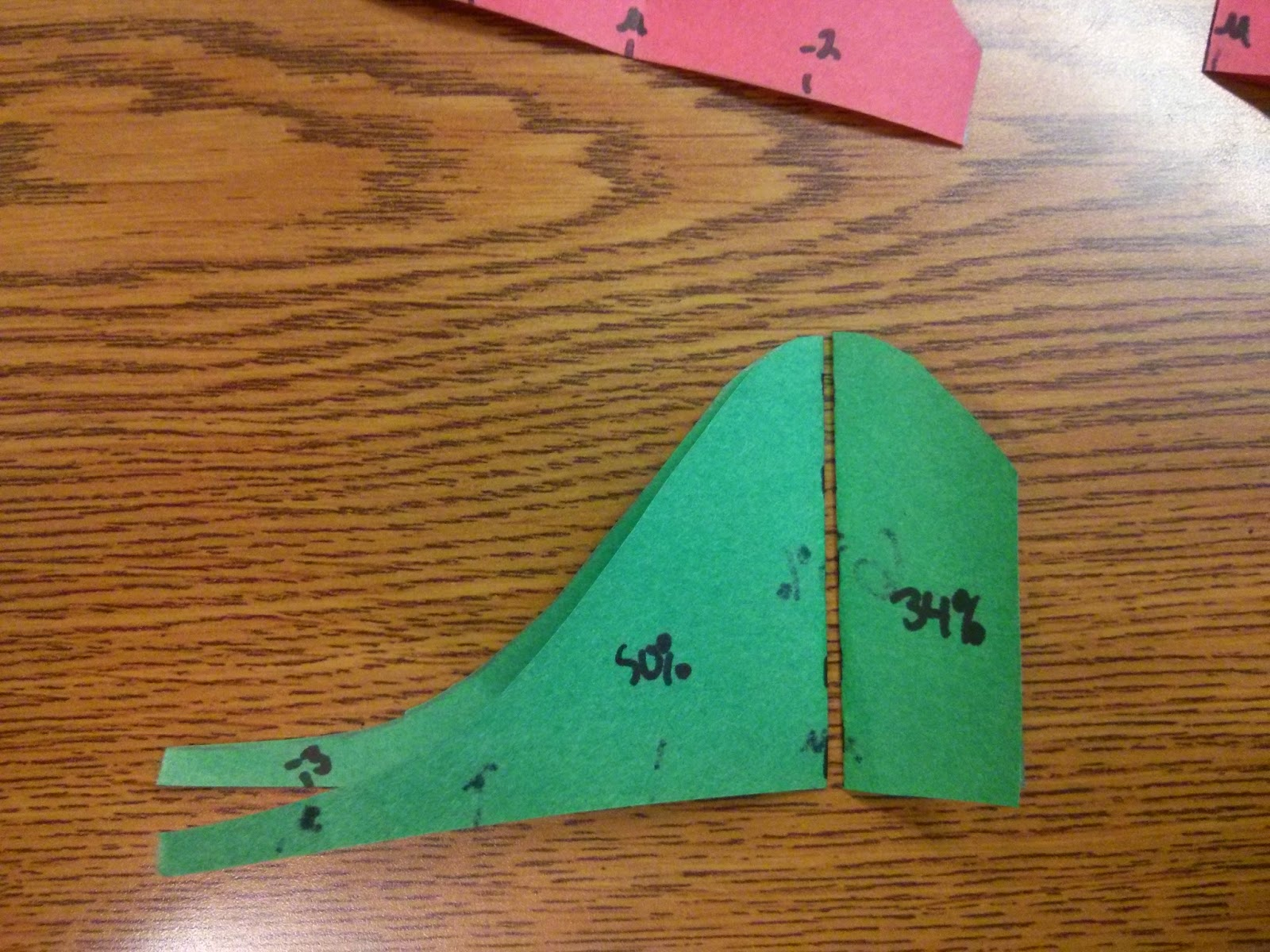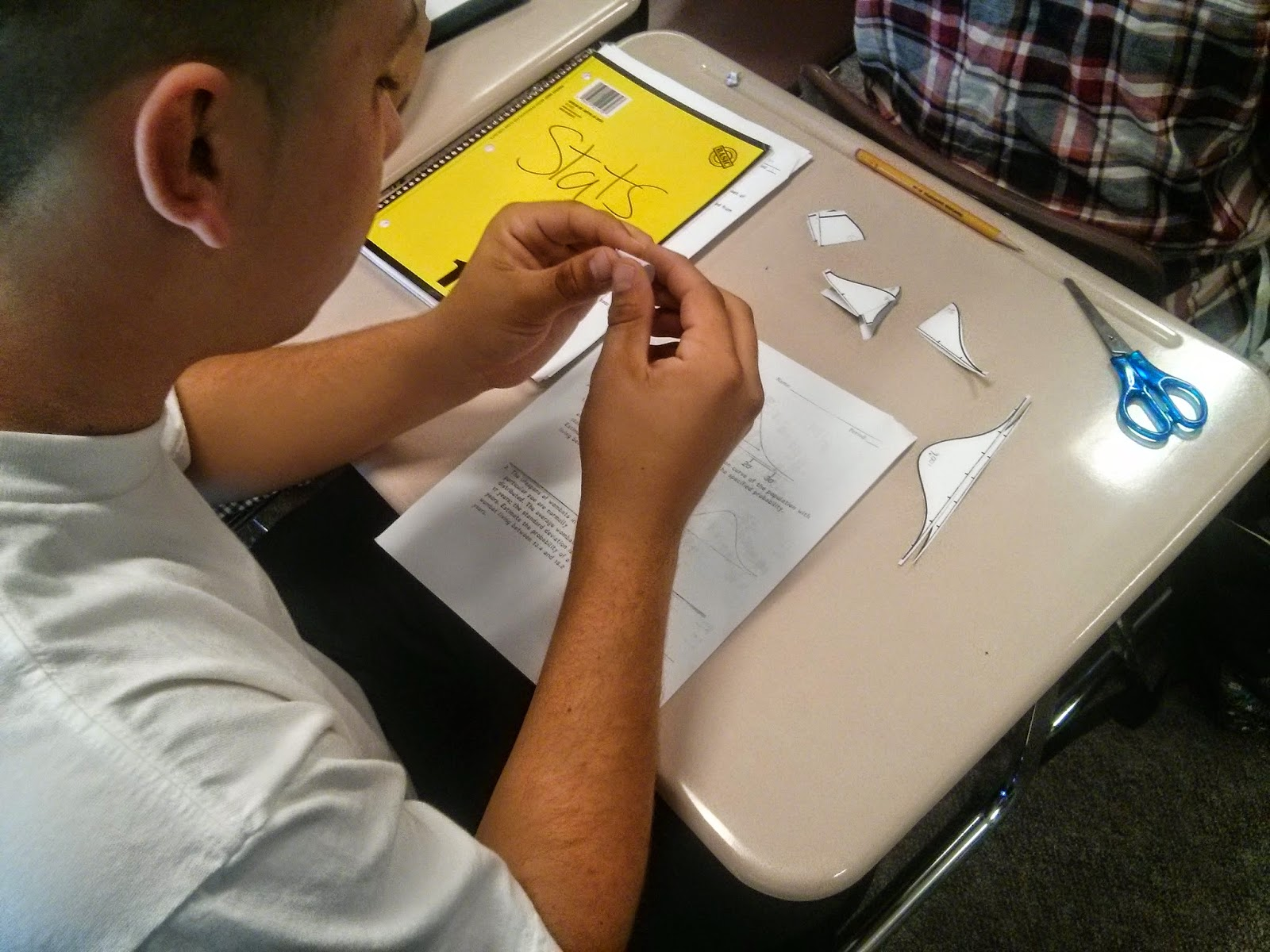# Empirical Rule Manipulatives

I put together a manipulative idea for teaching the Empirical Rule (68 - 95 - 99.7% Rule) to my Stats class. It is posted on here.This would fall under the Common Core standard S-ID.A.4:
Use the mean and standard deviation of a data set to fit it to a normal distribution and to estimate population percentages. Recognize that there are data sets for which such a procedure is not appropriate. Use calculators, spreadsheets, and tables to estimate areas under the normal curve.

I give two sheets to students. If you have colored paper, like a red and a green, its helps with the visual. I had my students color them with two different colors. If paper is in short supply, it can be done with just one set. Students should then cut out the curves.On the front, each one should be labeled 100%, 99.7%, 95%, and 68%. Then fold them in half and on the backs, label each half of the backs: 50%, 49.35%, 47.5% and 34%. Now they can put them together and just add or subtract them. For example, the probability of data being below 1 standard deviation is the 50% below the mean, and half of the piece for 1 standard deviation above the mean: 50% + 34% = 84%.Now if the endpoints of the data are on the same side of the mean, then you need to subtract. In this case, data between 1 standard deviation and 2 standard deviation is 47.5% then take out the red 34%, 47.5% – 34% = 13.5%Here are some students working with them.I used the Khan Academy exercise on Empirical Rule. And these are the worksheets that they are working on.